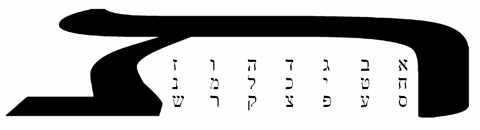LIBER TAV
VEL KABBALAE TRIVM LITERARUM
SVB FIGVRA CDThe Grades of Initiation: 1 א 8°=3□ The Magister Templi 8 ח 5°=6□ The Adeptus 60 ס 0°=0□ The Neophyte 1 + 8 + 60 = 69 = 3 x 2323 = חיה (Life) The Illusions: 2 ב The Ultimate Illusion 9 ט The Illusion of Force 70 ע The Illusion of Matter 2 + 9 + 70 = 81 = 3 x 2727 = זכ (Purity) The Functions of the 3 Orders: 3 ג Silence in Speech; Construction 10 י Silence; Preservation 80 פ Speech in Silence; Destruction 3 + 10 + 80 = 93 = 3 x 3131 = לא (Negation) The Unveilings: 4 ד The Supreme Unveiling (or Unveiling of Light) 20 כ The Unveiling of Life 90 צ The Unveiling of Love 4 + 20 + 90 = 114 = 3 x 3838 = זכאי (Innocent)38 x 11 = 418 Equilibrium: 5 ה On the Cubic Stone 30 ל On the Path 100 ק Among the Shells 5 + 30 + 100 = 135 = 3 x 4545 = מה (Formation) The Rituals of Initiation: 6 ו 8°=3□ Asar as Bull 40 מ 5°=6□ Asar as Man 200 ר 0°=0□ Asar as Sun 6 + 40 + 200 = 246 = 3 x 8282 = בעי (Prayer) The Ordeals of Initiation: 7 ז 8°=3□ Birth 50 נ 5°=6□ Death 300 ש 0°=0□ Resurrection 7 + 50 + 300 = 357 = 3 x 119119 = דמעה (Weeping)